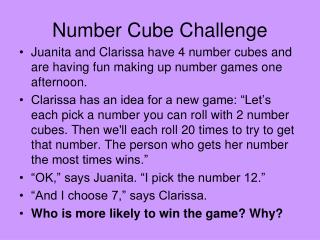DownloadDownload PresentationNumber Cube Challenge

# Number Cube Challenge

Télécharger la présentation## Number Cube Challenge

- - - - - - - - - - - - - - - - - - - - - - - - - - - E N D - - - - - - - - - - - - - - - - - - - - - - - - - - -
##### Presentation Transcript

1. Number Cube Challenge • Juanita and Clarissa have 4 number cubes and are having fun making up number games one afternoon. • Clarissa has an idea for a new game: “Let’s each pick a number you can roll with 2 number cubes. Then we'll each roll 20 times to try to get that number. The person who gets her number the most times wins.” • “OK,” says Juanita. “I pick the number 12.” • “And I choose 7,” says Clarissa. • Who is more likely to win the game? Why?

2. Number Cube Challenge • Clarissa is more likely to win because there are more ways to get 7 on the number cubes. • 1+6 = 7 • 2+5 = 7 • 3+4 = 7 • There is only one combination one the number cubes that will make 12. 6+6.

3. Area of Triangles and Trapezoids Lesson 11-5

4. 5 in 4 in • What is the area of the parallelogram? • Area = bxh • A = 20 in²

5. A = 20 in² • What happened to the parallelogram when we cut it in half? • We now have two triangles. • What would the area of one triangle be????????? • What could we use as a formula to find the area of a triangle?

6. Triangles h b The area of a triangle is half of the area of a parallelogram. A = ½ bh

7. Find the Area of the Triangle • A = ½ bh • A = ½ (6.5m) x (10m) • A = ½ (65m) • A = 32.5 m² 10m 6.5m

8. Find the Area of the Triangle • A = ½ bh • A = ½ (14ft) x (11ft) • A = ½ (154ft) • A = 77 ft² 14ft 11ft

9. Find the Area of the Triangle • A = ½ bh • A = ½ (8mm) x (9.6mm) • A = ½ (76.8mm) • A = 38.4 mm² 8mm 9.6mm

10. Trapezoids b 1 h b 2 • A trapezoid has two bases, b and b . The height (h) of a trapezoid is the distance between the bases. • A = ½ h( b + b ) 1 2 1 2

11. Find the Area of the Trapezoid 12 in 7 in • A = ½ h( b + b ) • A = ½ (7in)(5in +12in) • A = ½ (7in)(17in) • A = ½ (119in) • A = 59.5 in² 1 2 5 in

12. Find the Area of the Trapezoid 7 ft 8 ft • A = ½ h( b + b ) • A = ½ (8ft)(7ft +15.6ft) • A = ½ (8ft)(22.6ft) • A = ½ (180.8ft) • A = 90.4 ft² 1 2 15.6 ft

13. Triangles h b The area of a triangle is half of the area of a parallelogram. A = ½ bh

14. Trapezoids b 1 h b 2 • A trapezoid has two bases, b and b . The height (h) of a trapezoid is the distance between the bases. • A = ½ h( b + b ) 1 2 1 2

15. Don’t Forget • Homework: Lesson 11-5 # 2-14 evens